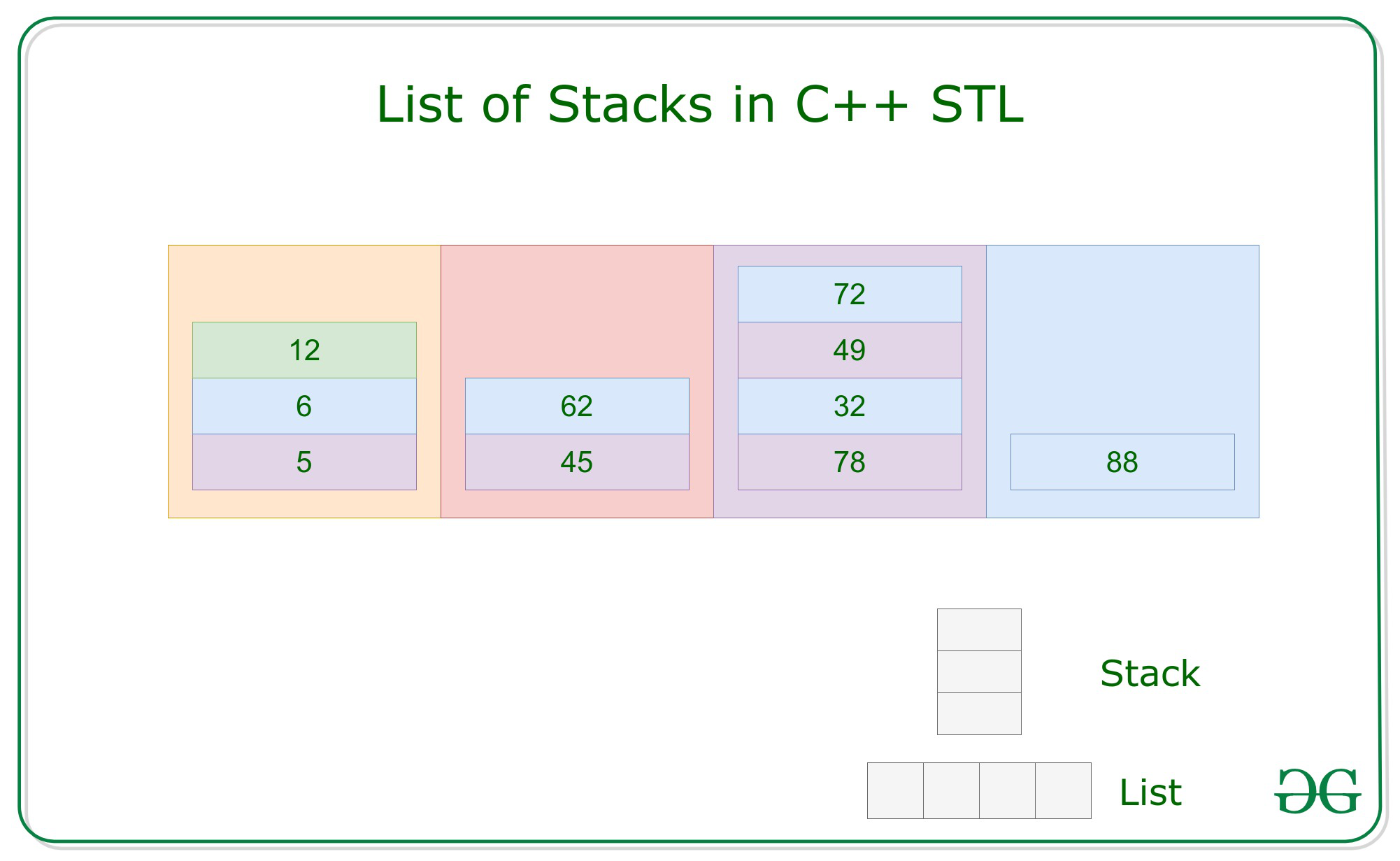# List of Stacks in C++ STL

Prerequisite: List, Stack

Lists are sequence containers that allow non-contiguous memory allocation. As compared to vector, list has slow traversal, but once a position has been found, insertion and deletion are quick.

Syntax:

list <Type> name_of_list;

Stack are a type of container adaptor with LIFO(Last In First Out) type of work, where a new element is added at one end and (top) an element is removed from that end only.

Syntax:

stack <Type> name_of_stack;

List of Stacks are a type of container which have a series of stacks, this is a two-dimensional container where N rows of list and M column of stacks, size of both dimension is non-fixed. which can be traversed and accessed using iterators.

Syntax:

list <stack <Type> > name_of_container(size);
where size is optionalExample:

list <stack <int> > ls(10);
size of list of stacks is 10

Insertion: Insertion in the list of the stack is done using the push() function.

Example:

## C++

 `// Loop to push element ` `// into a stack ` `for` `    ``i in[0, n] ` `    ``{ ` `        ``s.push(i) ` `    ``} ` ` `  `// Push Stack into the list ` `ls.push_back(s); `

Traversal: Traversal in a list of the stack is performed using iterators.

## C++

 `// Loop to iterate over list ` `for` `(iterator it = ls.begin(); ` `     ``it != ls.end(); it++) { ` ` `  `    ``// Stack of the List ` `    ``stack<``int``> ` `        ``st = *it; ` ` `  `    ``while` `(!st.empty()) { ` `        ``cout << st.top(); ` `        ``st.pop(); ` `    ``} ` `} `

Above code traverses list<int> ls at each index using starting iterators ls.begin() and ending iterator ls.end(). For accessing the element it uses (*it) as stack are pointers pointing to elements in list <stack <int> > ls.

Below is the program to illustrate the insertion and traversal in lists of stacks:

## C++

 `// C++ program for implementation ` `// of the lists of stack ` ` `  `#include ` `using` `namespace` `std; ` ` `  `// Function for printing the ` `// elements in a list ` `void` `showlist(list > ls) ` `{ ` ` `  `    ``// Traverse the list and ` `    ``// print row wise stack ` `    ``for` `(list >::iterator it1 ` `         ``= ls.begin(); ` `         ``it1 != ls.end(); ++it1) { ` ` `  `        ``// Copy rows in stack ` `        ``stack<``int``> it2 = *it1; ` ` `  `        ``// Print stack elements while ` `        ``// it is not empty ` `        ``while` `(!it2.empty()) { ` `            ``cout << it2.top() << ``" "``; ` `            ``it2.pop(); ` `        ``} ` `        ``cout << endl; ` `    ``} ` `} ` ` `  `// Driver Code ` `int` `main() ` `{ ` `    ``// List of stacks ` `    ``list > ls; ` ` `  `    ``// Insert rows in list ` `    ``for` `(``int` `i = 0; i < 10; ++i) { ` `        ``// Insert element in ` `        ``// stack as column ` `        ``stack<``int``> s; ` ` `  `        ``for` `(``int` `j = i; j < 10; j++) { ` `            ``s.push(j); ` `        ``} ` ` `  `        ``ls.push_back(s); ` `    ``} ` ` `  `    ``cout << ``"List of stack is : \n"``; ` `    ``showlist(ls); ` ` `  `    ``return` `0; ` `} `

Output:

```List of stack is :
9 8 7 6 5 4 3 2 1 0
9 8 7 6 5 4 3 2 1
9 8 7 6 5 4 3 2
9 8 7 6 5 4 3
9 8 7 6 5 4
9 8 7 6 5
9 8 7 6
9 8 7
9 8
9
```

Rated as one of the most sought after skills in the industry, own the basics of coding with our C++ STL Course and master the very concepts by intense problem-solving.

My Personal Notes arrow_drop_upStrategy Path planning and Destination matters in success No need to worry about in between temporary failures

If you like GeeksforGeeks and would like to contribute, you can also write an article using contribute.geeksforgeeks.org or mail your article to contribute@geeksforgeeks.org. See your article appearing on the GeeksforGeeks main page and help other Geeks.

Please Improve this article if you find anything incorrect by clicking on the "Improve Article" button below.